# Free Vibration of Conservative Single Degree of Freedom Systems (Part - 2) Civil Engineering (CE) Notes | EduRev

## Civil Engineering (CE) : Free Vibration of Conservative Single Degree of Freedom Systems (Part - 2) Civil Engineering (CE) Notes | EduRev

The document Free Vibration of Conservative Single Degree of Freedom Systems (Part - 2) Civil Engineering (CE) Notes | EduRev is a part of the Civil Engineering (CE) Course Introduction to Dynamics and Vibrations- Notes, Videos, MCQs.
All you need of Civil Engineering (CE) at this link: Civil Engineering (CE)

5.2.4 Calculating the number of degrees of freedom (and natural frequencies) of a system

When you analyze the behavior a system, it is helpful to know ahead of time how many vibration frequencies you will need to calculate. There are various ways to do this. Here are some rules that you can apply:

The number of degrees of freedom is equal to the number of independent coordinates required to describe the motion. This is only helpful if you can see by inspection how to describe your system. For the spring-mass system in the preceding section, we know that the mass can only move in one direction, and so specifying the length of the spring s will completely determine the motion of the system. The system therefore has one degree of freedom, and one vibration frequency. Section 5.6 provides several more examples where it is fairly obvious that the system has one degree of freedom.

For a 2D system, the number of degrees of freedom can be calculated from the equation

n = 3r + 2p - Nc

where:

r is the number of rigid bodies in the system
p is the number of particles in the system
Nc is the number of constraints (or, if you prefer, independent reaction forces) in the system

To be able to apply this formula you need to know how many constraints appear in the problem. Constraints are imposed by things like rigid links, or contacts with rigid walls, which force the system to move in a particular way. The numbers of constraints associated with various types of 2D connections are listed in the table below. Notice that the number of constraints is always equal to the number of reaction forces you need to draw on an FBD to represent the joint

 Roller joint1 constraint (prevents motion in one direction)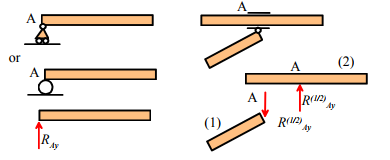Rigid (massless) link (if the link has mass, it should be represented as a rigid body)1 constraint (prevents relative motion parallel to link)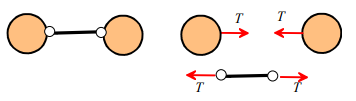Nonconformal contact (two bodies meet at a point)No friction or slipping: 1 constraint (prevents interpenetration)Sticking friction 2 constraints (prevents relative motion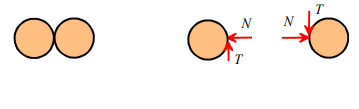Conformal contact (two rigid bodies meet along a line)No friction or slipping: 2 constraint (prevents interpenetration and rotation)Sticking friction 3 constraints (prevents relative motion)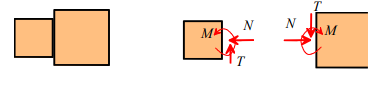Pinned joint (generally only applied to a rigid body, as it would stop a particle moving completely)2 constraints (prevents motion horizontally and vertically)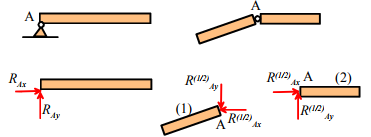Clamped joint (rare in dynamics problems, as it prevents motion completely)Can only be applied to a rigid body, not a particle3 constraints (prevents motion horizontally, vertically and prevents rotation)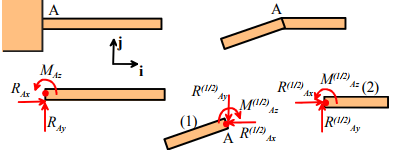For a 3D system, the number of degrees of freedom can be calculated from the equation

n = 6r + 3p - Nc

where the symbols have the same meaning as for a 2D system. A table of various constraints for 3D problems is given below.

 Pinned joint(5 constraints – prevents all motion, and prevents rotation about two axes)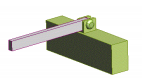Roller bearing(5 constraints – prevents all motion, and prevents rotation about two axes)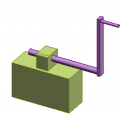Sleeve (4 constraints – prevents motion in two directions, and prevents rotation about two axes)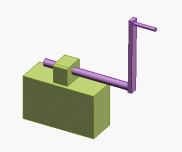Swivel joint 4 constraints (prevents all motion, prevents rotation about 1 axis)Ball and socket joint 3 constraints – prevents all motion.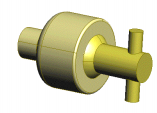Nonconformal contact (two rigid bodies meet at a point)No friction or slipping: 1 constraint (prevents interpenetration)Sticking friction 3 constraints, possibly 4 if friction is sufficient to prevent spin at contact)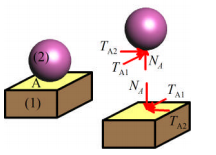Conformal contact (two rigid bodies meet over a surface)No friction or slipping: 3 constraints: prevents interpenetration and rotation about two axes.Sticking: 6 constraints: prevents all relative motion and rotation.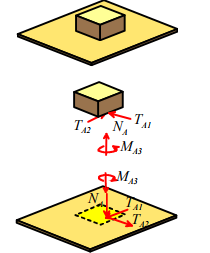Clamped joint (rare in dynamics problems, as it prevents all motion)6 constraints (prevents all motion and rotation)5.2.4 Calculating natural frequencies for 1DOF conservative systems

In light of the discussion in the preceding section, we clearly need some way to calculate natural frequencies for mechanical systems. We do not have time in this course to discuss more than the very simplest mechanical systems. We will therefore show you some tricks for calculating natural frequencies of 1DOF, conservative, systems. It is best to do this by means of examples.

Example 1: The spring-mass system revisited

Calculate the natural frequency of vibration for the system shown in the figure. Assume that the contact between the block and wedge is frictionless. The spring has stiffness k and unstretched length L0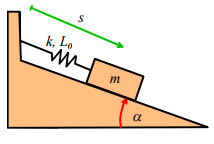Our first objective is to get an equation of motion for s. We could do this by drawing a FBD, writing down Newton’s law, and looking at its components. However, for 1DOF systems it turns out that we can derive the EOM very quickly using the kinetic and potential energy of the system.

The potential energy and kinetic energy can be written down as: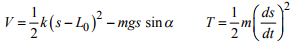(The second term in V is the gravitational potential energy – it is negative because the height of the mass decreases with increasing s). Now, note that since our system is conservative

T + V = constant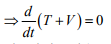Differentiate our expressions for T and V (use the chain rule) to see that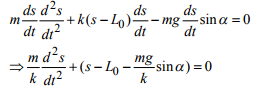Finally, we must turn this equation of motion into one of the standard solutions to vibration equations. Our equation looks very similar to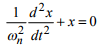Thus let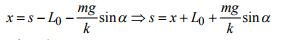and substitute into the equation of motion: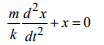By comparing this with our equation we see that the natural frequency of vibration is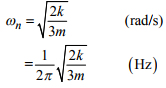Summary of procedure for calculating natural frequencies:

(1) Describe the motion of the system, using a single scalar variable (In the example, we chose to describe motion using the distance s);
(2) Write down the potential energy V and kinetic energy T of the system in terms of the scalar variable;
(3) Use d/dt (T + V) = 0 to get an equation of motion for your scalar variable;
(4) Arrange the equation of motion in standard form;
(5) Read off the natural frequency by comparing your equation to the standard form.

Example 2: A nonlinear system.

We will illustrate the procedure with a second example, which will demonstrate another useful trick.

Find the natural frequency of vibration for a pendulum, shown in the figure. We will idealize the mass as a particle, to keep things simple.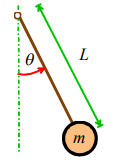We will follow the steps outlined earlier:
(1) We describe the motion using the angle θ
(2) We write down T and V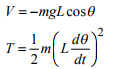(if you don’t see the formula for the kinetic energy, you can write down the position vector of the mass as r = L sinθi - L cosθj , differentiate to find the velocity: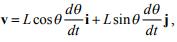and then compute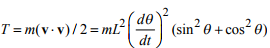and use a trig identity. You can also use the circular motion formulas, if you prefer).

(3) Differentiate with respect to time: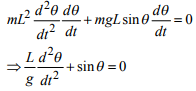(4) Arrange the EOM into standard form. Houston, we have a problem. There is no way this equation can be arranged into standard form. This is because the equation is nonlinear (sinθ is a nonlinear function of θ). There is, however, a way to deal with this problem. We will show what needs to be done, summarizing the general steps as we go along.

(i) Find the static equilibrium configuration(s) for the system.

If the system is in static equilibrium, it does not move. We can find values of  for which the system is in static equilibrium by setting all time derivatives of  in the equation of motion to zero, and then solving the equation. Here,

sinq θo = 0 ⇒ θ0 = 0,π , 2π ...

Here, we have used θ0 to denote the special values of θ for which the system happens to be in static equilibrium. Note that θ0 is always a constant.

(ii) Assume that the system vibrates with small amplitude about a static equilibrium configuration of interest.

To do this, we let θ = θ0 + x , where x << 1 .

Here, x represents a small change in angle from an equilibrium configuration.. Note that x will vary with time as the system vibrates. Instead of solving for q , we will solve for x. Before going on, make sure that you are comfortable with the physical significance of both x and θ0 .

(iii) Linearize the equation of motion, by expanding all nonlinear terms as Taylor Maclaurin series about the equilibrium configuration.

We substitute for θ in the equation of motion, to see that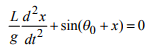(Recall that θ0 is constant, so its time derivatives vanish)

Now, recall the Taylor-Maclaurin series expansion of a function f(x) has the form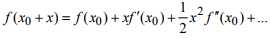where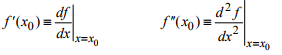Apply this to the nonlinear term in our equation of motion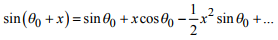Now, since x<<1, we can assume that  xn<<x , and so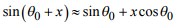Finally, we can substitute back into our equation of motion, to obtain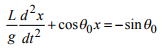(iv) Compare the linear equation with the standard form to deduce the natural frequency.

We can do this for each equilibrium configuration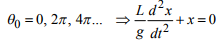whence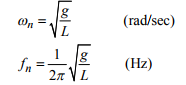Note that all these values of θ0 really represent the same configuration: the mass is hanging below the pivot. We have rediscovered the well-known expression for the natural frequency of a freely swinging pendulum.

Next, try the remaining static equilibrium configuration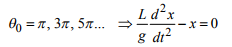If we look up this equation in our list of standard solutions, we find it does not have a harmonic solution. Instead, the solution is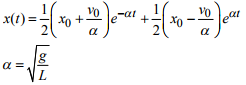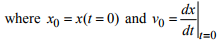Thus, except for some rather special initial conditions, x increases without bound as time increases. This is a characteristic of an unstable mechanical system.

If we visualize the system with θ0 = π , we can see what is happening. This equilibrium configuration has the pendulum upside down!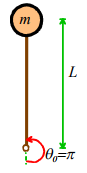No wonder the equation is predicting an instability…

Here is a question to think about. Our solution predicts that both x and dx/dt become infinitely large. We know that a real pendulum would never rotate with infinite angular velocity. What has gone wrong?

Example 3: We will look at one more nonlinear system, to make sure that you are comfortable with this procedure. Calculate the resonant frequency of small oscillations about the equilibrium configuration θ = 0 for the system shown. The spring has stiffness k and unstretched length L0 .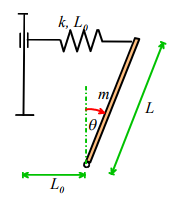We follow the same procedure as before.

The potential and kinetic energies of the system are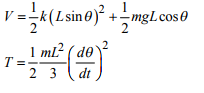Hence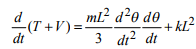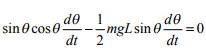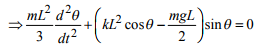Once again, we have found a nonlinear equation of motion. This time we know what to do. We are told to find natural frequency of oscillation about θ = 0 , so we don’t need to solve for the equilibrium configurations this time. We set θ = 0 + x , with x <<1 and substitute back into the equation of motion: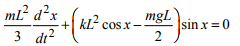Now, expand all the nonlinear terms (it is OK to do them one at a time and then multiply everything out. You can always throw away all powers of x greater than one as you do so)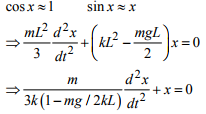If you prefer, you can use Mathematica to do the Taylor series expansion: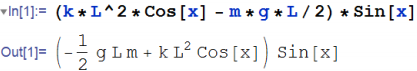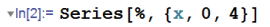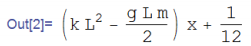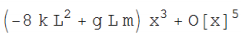(The number 4 in the ‘series’ command specifies the highest power of x that should appear in the expansion). We now have an equation in standard form, and can read off the natural frequency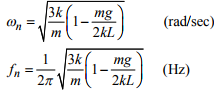Question: what happens for mg > 2kL ?

Example 3: A system with a rigid body (the KE of a rigid body will be defined in the next section of the course – just live with it for now!).

Calculate the natural frequency of vibration for the system shown in the figure. Assume that the cylinder rolls without slip on the wedge. The spring has stiffness k and unstretched length L0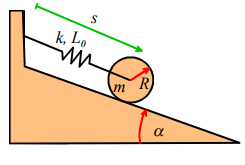Our first objective is to get an equation of motion for s. We do this by writing down the potential and kinetic energies of the system in terms of s.

The potential energy is easy: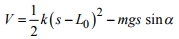The first term represents the energy in the spring, while second term accounts for the gravitational potential energy.

The kinetic energy is slightly more tricky. Note that the magnitude of the angular velocity of the disk is related to the magnitude of its translational velocity byThus, the combined rotational and translational kinetic energy follows as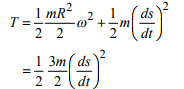Now, note that since our system is conservative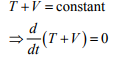Differentiate our expressions for T and V to see that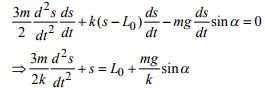The last equation is almost in one of the standard forms given on the handout, except that the right hand side is not zero. There is a trick to dealing with this problem – simply subtract the constant right hand side from s, and call the result x. (This only works if the right hand side is a constant, of course). Thus let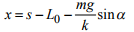and substitute into the equation of motion: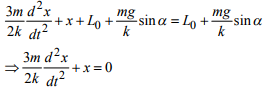This is now in the form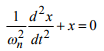and by comparing this with our equation we see that the natural frequency of vibration is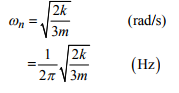Offer running on EduRev: Apply code STAYHOME200 to get INR 200 off on our premium plan EduRev Infinity!

## Introduction to Dynamics and Vibrations- Notes, Videos, MCQs

20 videos|53 docs

,

,

,

,

,

,

,

,

,

,

,

,

,

,

,

,

,

,

,

,

,

;